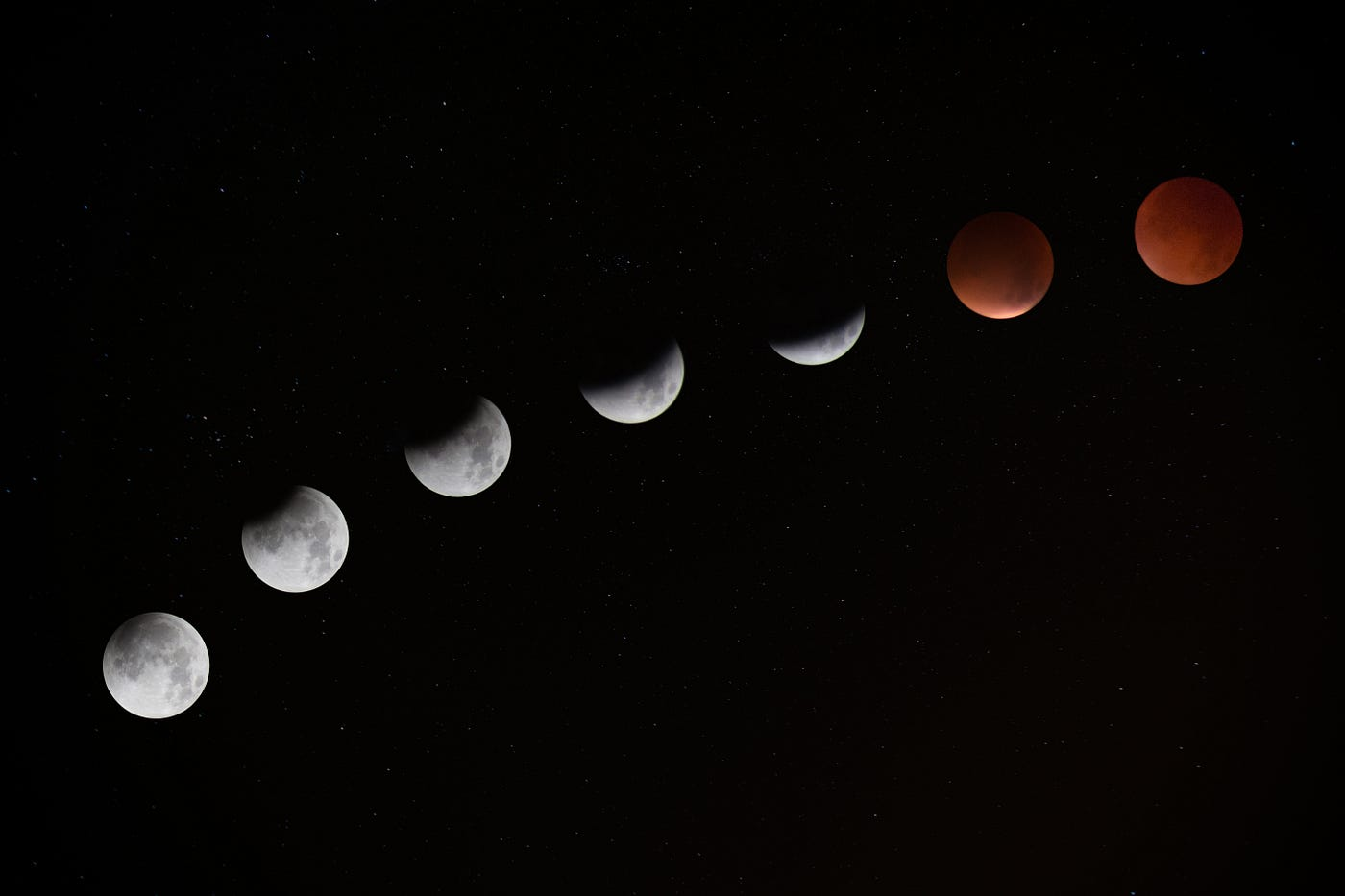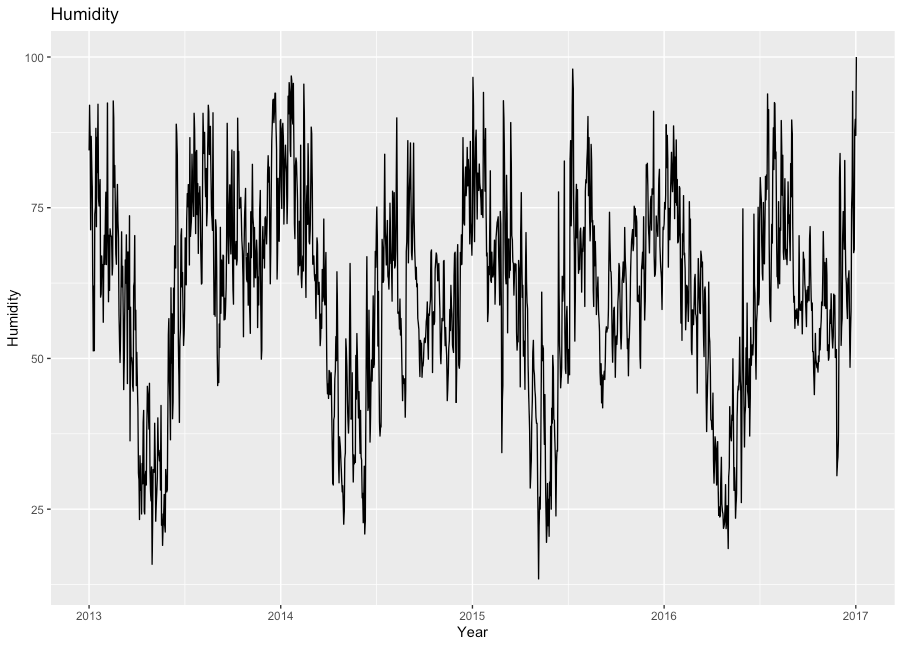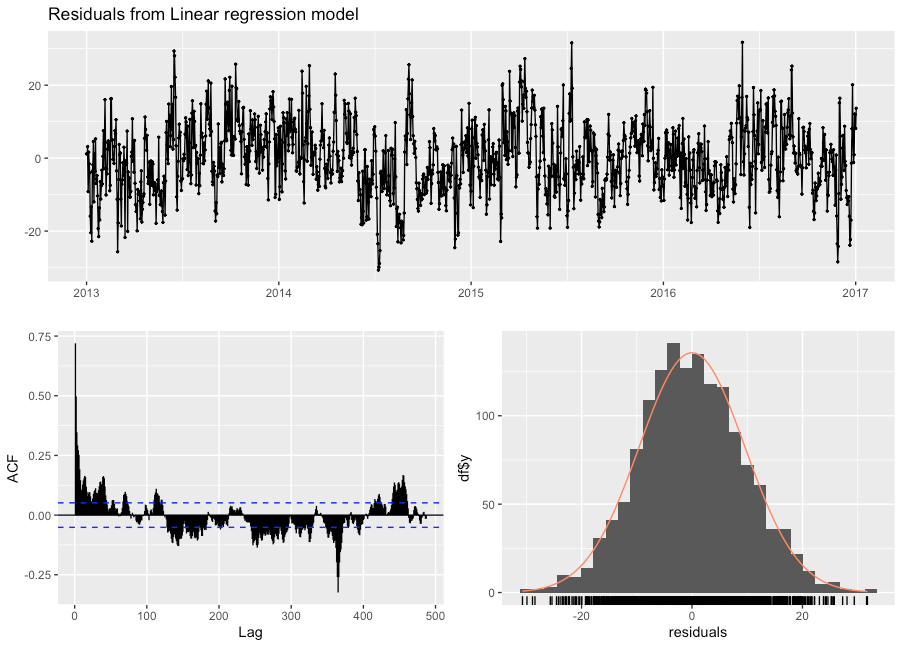# Time-series analysis — Part 1Photo by Jake Hills on Unsplash

Time series data is ordered data points collected at regular intervals. It can also be described as discrete-time data. Time-series data is ubiquitous and is used in various fields like statistics, finance, earthquake prediction, astronomy e.t.c. Time-series analysis is a specific way of analyzing time-series data. The most common form of time-series data analysis is forecasting. It is used in making scientifically informed predictions based on the historical information collected.

`# reading the humidity time-series data from kaggle #library(ts_plot)library(ggplot2)library(ggfortify)library(fpp2)library(forecast)library(GGally)data <- read.csv('DailyDelhiClimateTrain.csv')df <- ts(data, start=c(2013,1,1), frequency=365)data1 <- read.csv('DailyDelhiClimateTest.csv')df1 <- ts(data1, start=c(2017,1,1), frequency=365)## plotting humidity ##autoplot(df[,"humidity"]) +  ggtitle("Humidity") + xlab("Year") +  ylab("Humidity")`Variation of humidity over time (Kaggle data)

Time-series data can be decomposed into seasonality, trend, cyclic and irregular.

Seasonality: Seasonal effect occurs in the data due to factors like the day or day of the year. The seasonal effects have a fixed frequency.

Trend: Trend exists in the data when there is a long-term increase or decrease in data.

The above graph displaying humidity with time has a trend and seasonal component.

Cyclic: Cyclic is similar to seasonality, with the difference being that the frequency is not fixed.

We will be using a time-series data of humidity in New Delhi over a period of four years to conduct our analysis. There are various simple methods to forecast humidity data:

1. Average method

In this method, we forecast that all future values are equal to the average of all historical values.

2. Naive method

In this method, we forecast that all future values are equal to be the last value of the observation.

3. Seasonal Naive method

This method is in case of highly seasonal data. We set the the forecast values to be equal to the last value observed in the same season of the year.

Here, m = the seasonal period, k = integer part of (h-1)/m and h is the number of time periods complete between T and T+h.

4. Drift method

This method allows the forecast to decrease or increase over time and this change is equal to the average change in the historical data.

`#### naive, seasonal naive, mean method and drift method ##autoplot(df[,'humidity']) +  autolayer(meanf(df[,'humidity'], h=365), series="Mean", PI=FALSE) +  autolayer(naive(df[,'humidity'], h=365), series="Naïve", PI=FALSE) +  autolayer(rwf(df[,'humidity'], drift=TRUE, h=365), series="Drift", PI=FALSE) +  autolayer(snaive(df[,'humidity'], h=365), series="Seasonal naïve", PI=FALSE) +  autolayer(df1[,'humidity'], series="Actual", PI=FALSE) +  ggtitle("Comparison of actual humidity with humidity forecast") + xlab("Year") +   ylab("Humidity") + guides(colour=guide_legend(title="Forecast"))`

We are now going to use Linear regression model to analyze our time-series data. We will decompose our humidity data into season and trend.

`## linear regression model ##ts.model <- tslm(df[,'humidity'] ~ trend + season)## plotting data forecast ##autoplot(df[,'humidity']) +  autolayer(forecast(ts.model, h = 100), series = "Linear Regression") +  autolayer(df1[,'humidity'], series="Actual", PI=FALSE) +  ggtitle("Comparison of actual humidity with humidity forecast") + xlab("Year") +   ylab("Megalitres") + guides(colour=guide_legend(title="Forecast"))`
`checkresiduals(ts.model)`Residual analysis of the linear regression model

The residual of the linear regression model is clearly normal but from the ACF it is clear that the error term is dependent on the lagged and hence, there is auto-correlation. In this case, we will have to employ other more complex methods like Holt’s method and auto-regressive integrated moving average (ARIMA) models. We will dive deep into the these models in part 2 3 of this article series.

--

--